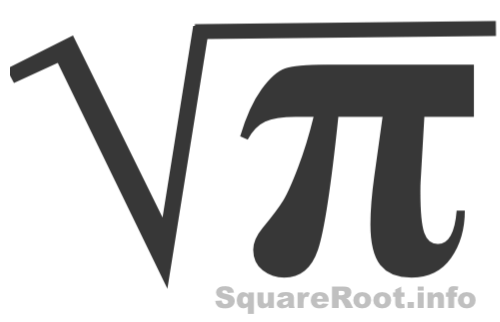Square Root of Pi (√π)Here we will define, explain, and show you how to calculate the square root of Pi. First, note that Pi or Pie as it is sometimes referred to can be written with the Pi symbol: π

Pi (π) is not a perfect square, but an irrational decimal number that cannot be simplified. Pi goes on indefinitely, so obviously it is not possible to list the entire Pi. However, below is the beginning of Pi.

3.1415926535

Square root of Pi definition
The square root of Pi in mathematical form is written with the radical sign like this: √π. We call this the square root of Pi in radical form. The square root of Pi is a quantity (q) that when multiplied by itself will equal Pi.

√π = q × q = q2

How to calculate the square root of Pi with a calculator
The easiest and most boring way to calculate the square root of Pi is to use your calculator! Simply press π followed by √x to get the answer. We did that with our calculator and got the following answer with 9 decimal numbers:

√π ≈ 1.772453851

How to calculate the square root of Pi with a computer
If you are using a computer that has Excel or Numbers, then you can enter SQRT(PI) in a cell to get the square root of Pi. Below is the result we got with 14 decimals. We call this the square root of Pi in decimal form.

SQRT(PI) ≈ 1.77245385090552

What is the square root of Pi rounded?
The square root of Pi rounded to the nearest tenth, means that you want one digit after the decimal point. The square root of Pi rounded to the nearest hundredth, means that you want two digits after the decimal point. The square root of Pi rounded to the nearest thousandth, means that you want three digits after the decimal point.

10th: √π ≈ 1.8

100th: √π ≈ 1.77

1000th: √π ≈ 7.772

What is the square root of Pi as a fraction?
Like we said above, since the square root of Pi is an irrational number, we cannot make it into an exact fraction. However, we can make it into an approximate fraction using the square root of Pi rounded to the nearest hundredth.

π
≈ 1.77/1
≈ 177/100
≈ 1 77/100

What is the square root of Pi written with an exponent?
All square roots can be converted to a number (base) with a fractional exponent. The square root of Pi is no exception. Here is the rule and the answer to "the square root of Pi converted to a base with an exponent":

b = b½

π = π½

How to find the square root of Pi by long division method
Here we will show you how to calculate the square root of Pi using the long division method. We will calculate the square root of Pi using the first five digits of Pi which is 3.1415.

Step 1)
Set up 3.1415 in pairs of two digits from right to left like this:

 3 14 15

Step 2)
Starting with the first set: the largest perfect square less than or equal to 3 is 1, and the square root of 1 is 1. Therefore, put 1 on top and 1 at the bottom like this:

 1 3 14 15 1

Step 3)
Calculate 3 minus 1 and put the difference below. Then move down the next set of numbers.

 1 3 14 15 1 2 14

Step 4)
Double the number in green on top: 1 × 2 = 2. Then, use 2 and the bottom number to make this problem:

2? × ? ≤ 214

The question marks are "blank" and the same "blank". With trial and error, we found the largest number "blank" can be is 7. Replace the question marks in the problem with 7 to get:

27 × 7 = 189.

Now, enter 7 on top, and 189 at the bottom:

 1 7 3 14 15 1 2 14 1 89

Step 5)
Calculate 214 minus 189 and put the difference below. Then move down the next set of numbers.

 1 7 3 14 15 1 2 14 1 89 25 15

Step 6)
Double the number in green on top: 17 × 2 = 34. Then, use 34 and the bottom number to make this problem:

34? × ? ≤ 2515

The question marks are "blank" and the same "blank". With trial and error, we found the largest number "blank" can be is 7. Now, enter 7 on top:

 1 7 7 3 14 15 1 2 14 1 89 0 25 15

That's it! The answer is on top. The square root of Pi with two digit decimal accuracy is 1.77. Did you notice that the last two steps repeat the previous two steps. You can add decimals by simply adding more sets of of numbers from Pi and repeating the last two steps over and over to get a more accurate answer.

Square Root of a Number
Please enter another number in the box below to get the square root of the number and other detailed information like you got for Pi on this page.